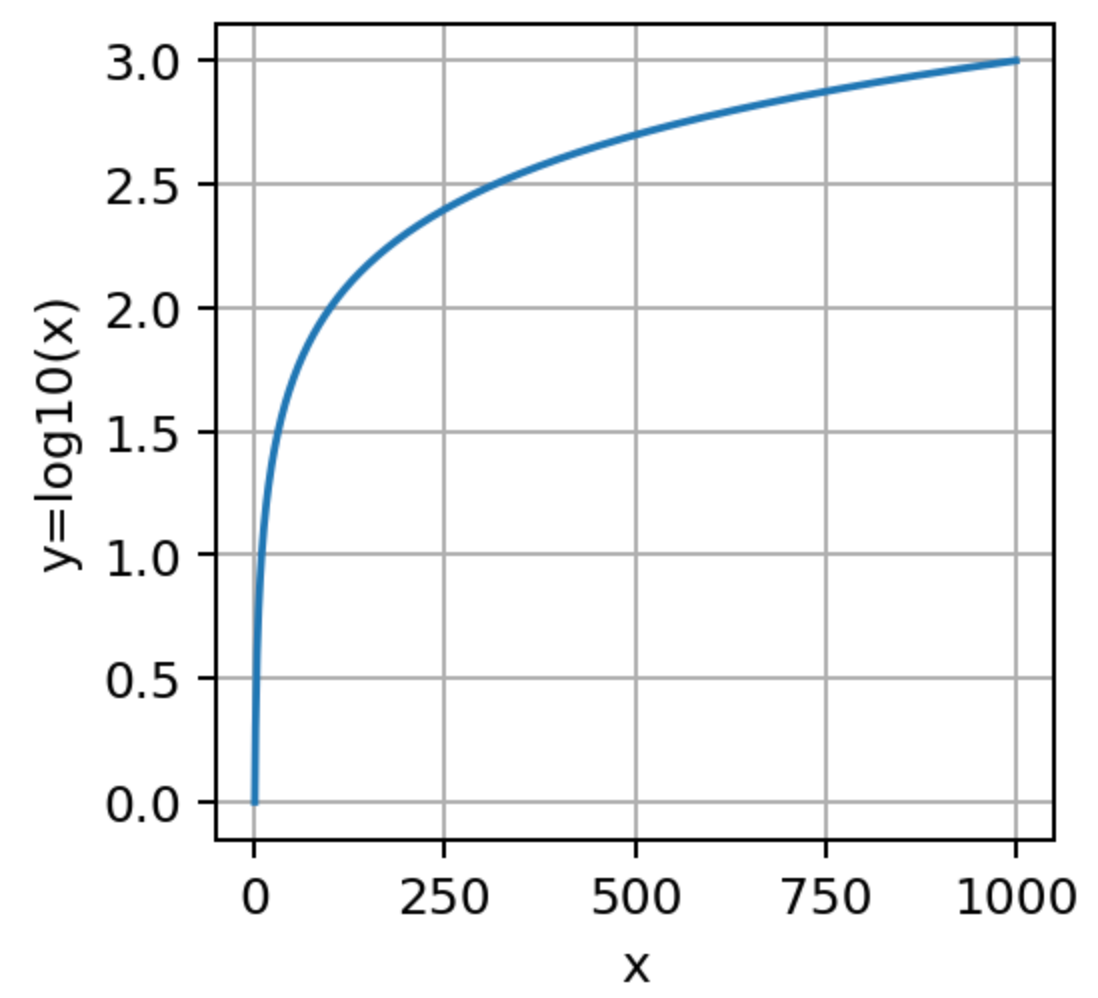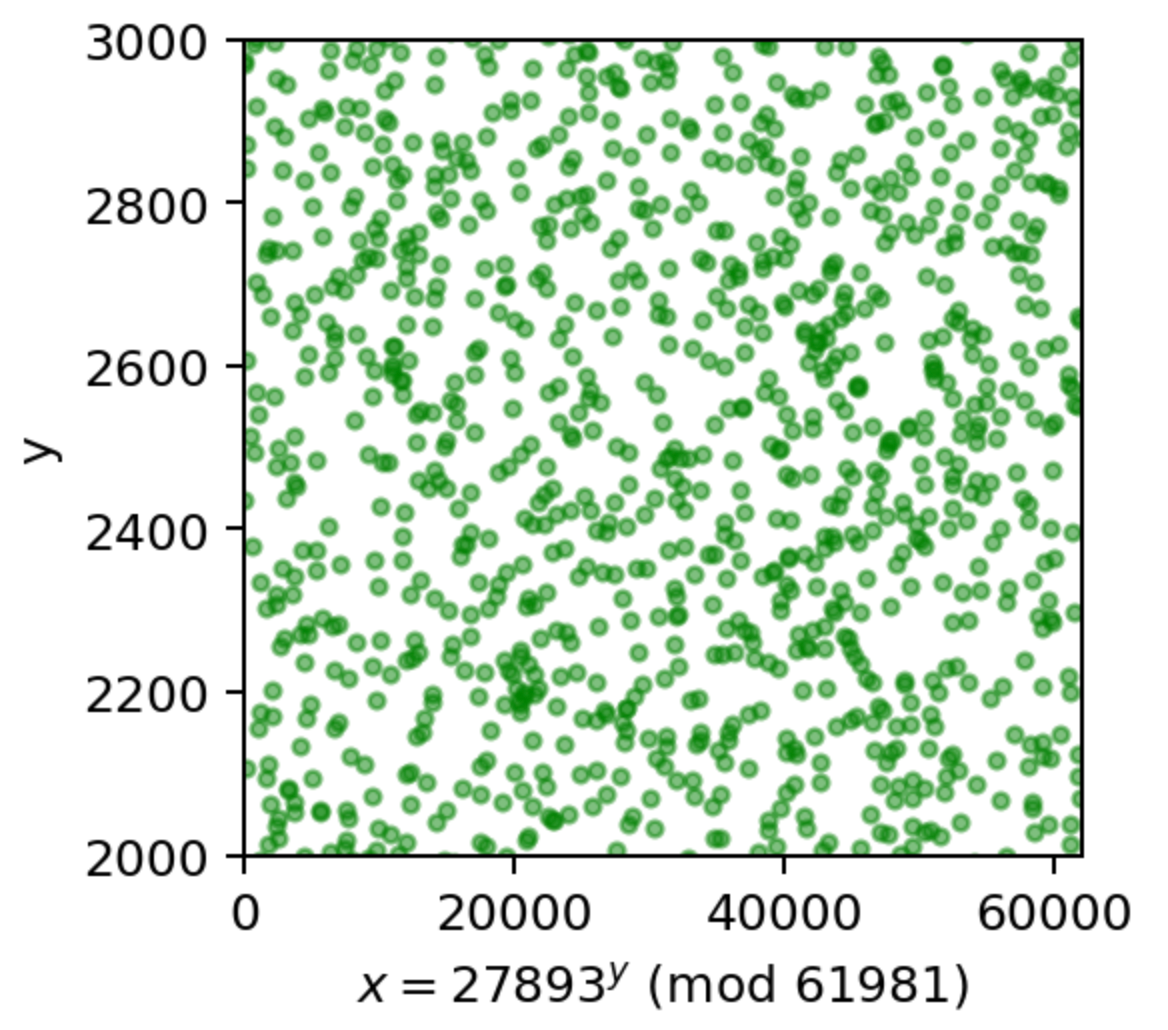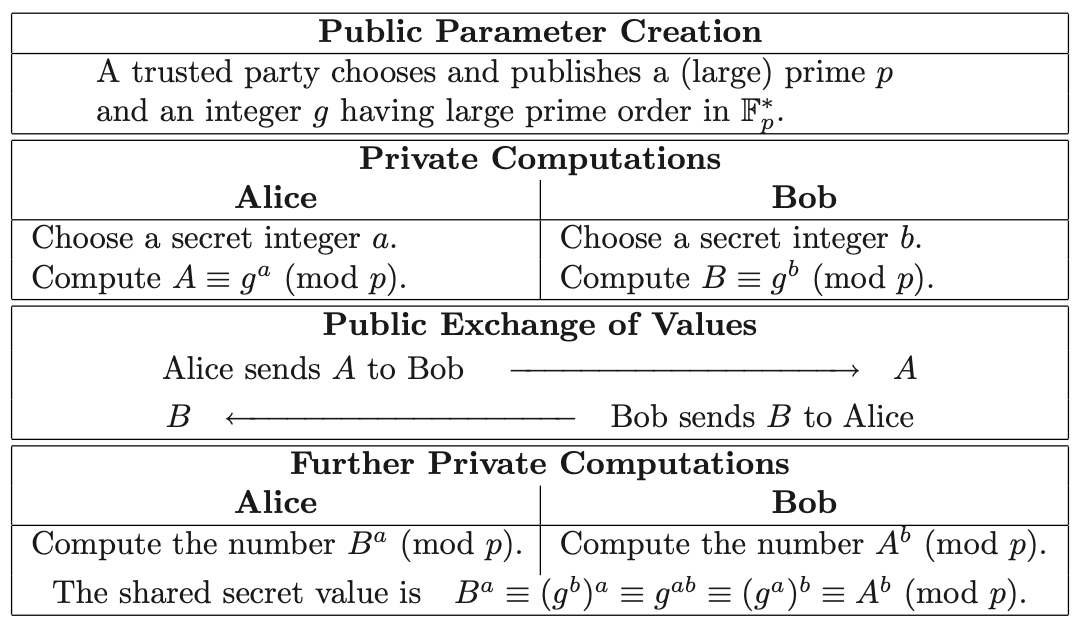# The discrete logarithm problem

Warning!. For this section, you may need to refresh an older post on algebra and modulo arithmetics from this series.logarithm base 10 as a function of x.Plot of x for several values of y for the group defined by p=61981 using the group generator element g=27893.

# How hard it is to break the discrete logarithm problem?

I hope I have convinced you that finding y in the DLP is a difficult task. But how hard is it?. Let’s try to break the DLP problem by randomly guessing the values of y. This is, given the triplet (x, g, p) we will randomly try different y until we find that x=g^y(mod p).

# The Diffie-Hellman key exchange

Now let’s get back to the main aim of this post, that is, to find a way for Alice and Bob to agree on a number when communicating through an insecure channel. For this we can exploit the difficulty to crack the DLP problem (see the book of Hoffstein, Pipher and Silverman). In the book you find a nice chart explaining the Diffie-Hellman key exchange:Diffie-Hellman key exchange. Table 2.2 from the book of Hoffstein Pipher and Silverman. A masterpiece, you must buy it!

# Takeaways

We have seen how difficult it is to compute discrete logarithms over modulo prime groups and how this can help us generate a common secret key in between two parties communicating through an insecure channel. That is the only ingredient we were missing for secure communication once we have a secure symmetric cipher.

--

--